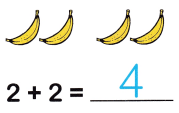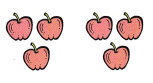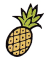All the solutions provided in McGraw Hill Math Grade 1 Answer Key PDF Chapter 2 Lesson 1 Addition Facts Through 6 as per the latest syllabus guidelines.

Write the sum.

Question 1.Question 2.1 + 4 = ___
Explanation:
There are 5 peaches in the given figure

Question 3.3 + 3 = ____
Explanation:
The sum of 3 apples and 3 apples is 6 apples
3 + 3 = 6

Question 4.2 + 1 = ____
Explanation:
2 + 1 = 3
The sum of 2 lemon and 1 lemon is 3 lemons.

Question 5.1 + 0 = ___